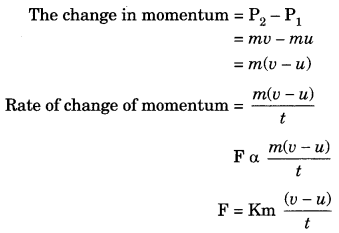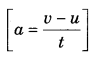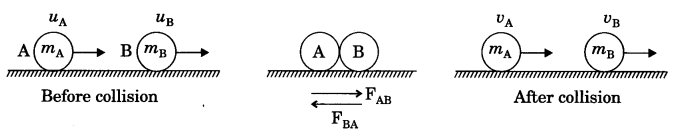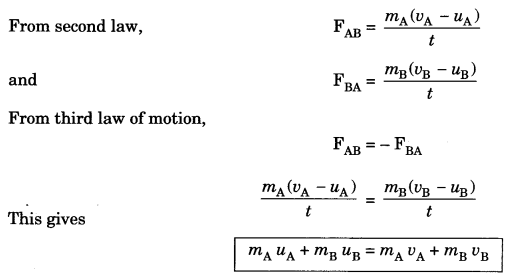On this page, you will find Force and Laws of Motion Class 9 Notes Science Chapter 9 Pdf free download. CBSE NCERT Class 9 Science Notes Chapter 9 Force and Laws of Motion will seemingly help them to revise the important concepts in less time.

## CBSE Class 9 Science Chapter 9 Notes Force and Laws of Motion

### Force and Laws of Motion Class 9 Notes Understanding the Lesson

1. Force
It is entity which when applied on a body changes or tends to change a body’s

• state of rest
• state of uniform motion
• direction of motion
•  shape

2. Balanced forces
When a number of forces acting simultaneously on a body do not bring about any change in state of rest or of uniform motion along a straight line, then forces acting on a body are said to be balanced forces.

3. Unbalanced forces
When a number of forces acting simultaneously on a body bring about a change in its state of rest or of uniform motion along a straight line, then these forces acting on the body are said to be unbalanced forces.

4. Newton’s first law of motion
An object remains in a state of rest or of uniform motion in a straight line unless compelled to change that state by an applied force.

5. Inertia
Inertia is the natural tendency of an object to resist a change in its state of motion or of rest.

6. The mass of an object is a measure of its inertia.
Examples:

• Passenger tends to fall backward, when a bus starts suddenly.
• Falling of fruits and leaves by a shaking tree.
• When a carpet is beaten with a stick, dust particles come out.
• When the card covering a glass tumbler is flicked with the finger coin placed over it falls in the tumbler.

7. Momentum (P)

• Momentum gives an idea about the quantity of motion contained in a body.
• The momentum (P) of an object is defined as the product of its mass (m) and velocity (v).
P = mv
• Momentum is vector quantity and its unit is kg ms-1.

8. Second law of motion
The second law of motion states that the rate of change of momentum of an object is proportional to the applied unbalanced force in the direction of force.

9. Mathematical formulation of second law of motion
Suppose an object of mass, m is moving along a straight line with an initial velocity, v. It is uniformly accel-erated to velocity, v in time, t by application of constant force F throughout the time, t. The initial and final momentum of the object will be, P1 = mu and P2 = mv
respectively.∴ F =ma
K is proportionality constant and the value So,
SI unit of force is newton.

10. First law of motion can be stated from the second law
F = ma
$$F=\frac{m(v-u)}{t}$$
Ft = mv – mu
when F = 0, v – u. This means that the object will continue moving with uniform velocity, u throughout the time, t. If u is zero then v will also be zero. That is, the object will remain at rest.

11. Example of second law of motion:
As we know that $$\text { F } \alpha \frac{1}{t}$$

• A cricket players lowers his hand while catching the ball to increase the time so that impact of force decreases.
• A karate player can break a pile of tiles with a single blow of his hand. This is because, as time decreases impact of force increases.
• Vehicles are fitted with shockers. The shockers increase the time of transmission of the force of the jerk to reach the floor of the vehicle. Hence less jerk is experienced by the passengers.

12. Third law of motion
According to the third law of motion, every action there is an equal and opposite reaction and they act on two different bodies.

Example of third law of motion:

• Recoiling of gun.
• When a man jumps out from a boat, the boat moves backward.

13. Conservation of momentum
In an isolated system (where there is no external force), the total momentum remains conserved.
mAuA + mBuB = mAvA + mBvB14. Derivation
Suppose two objects of mass mA and mB are travelling in the same direction along a straight line at different velocity uA and uB respectively, and there are no external unbalanced forces acting on them. Let uA > uB and two balls collide each other. During collision which last for a time t, ball A exerts force on ball B and ball B exerts force FBA on ball A. Suppose vA and i>B are the velocities after collision.15. Illustration of conservation of momentum

• Recoil of gun.
• Rocket propulsion.
• Inflated balloon lying on the surface of a floor moves forward when pierced with a pin.

### Class 9 Science Chapter 9 Notes Important Terms

Force: It is entity which when applied on a body changes or tends to change a body’s.

• state of rest
• state of uniform motion
• direction of motion
• shape

Balanced forces: When a number of forces acting simultaneously on a body do not bring about any change in state of rest or of uniform motion along a straight line, then forces acting on a body are said to be balanced forces.

Unbalanced forces: When a number of forces acting simultaneously on a body bring about change in its state of rest or of uniform motion along a straight line, then these forces acting on the body are said to be unbalanced forces.

Inertia: Inertia is the natural tendency of an object to resist a change in its state of motion or of rest.

Momentum: Momentum of a body is product of its mass and velocity.

Conservation of momentum: In an isolated system the total momentum remains conserved.